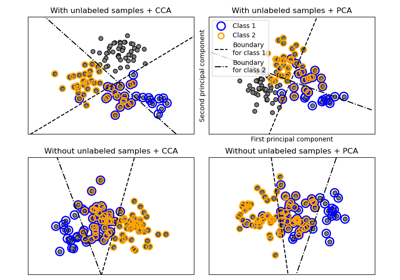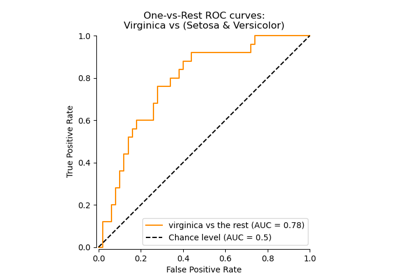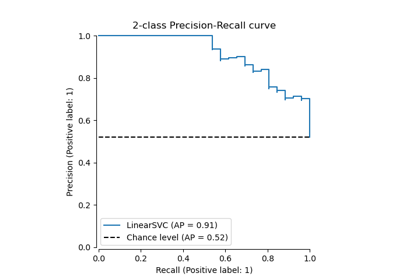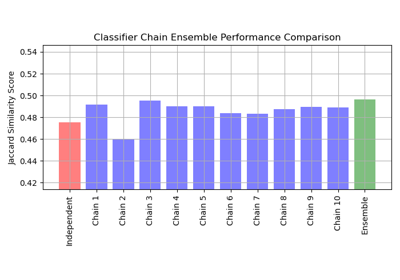# sklearn.multiclass.OneVsRestClassifier¶

class sklearn.multiclass.OneVsRestClassifier(estimator, *, n_jobs=None, verbose=0)[source]

One-vs-the-rest (OvR) multiclass strategy.

Also known as one-vs-all, this strategy consists in fitting one classifier per class. For each classifier, the class is fitted against all the other classes. In addition to its computational efficiency (only n_classes classifiers are needed), one advantage of this approach is its interpretability. Since each class is represented by one and one classifier only, it is possible to gain knowledge about the class by inspecting its corresponding classifier. This is the most commonly used strategy for multiclass classification and is a fair default choice.

OneVsRestClassifier can also be used for multilabel classification. To use this feature, provide an indicator matrix for the target y when calling .fit. In other words, the target labels should be formatted as a 2D binary (0/1) matrix, where [i, j] == 1 indicates the presence of label j in sample i. This estimator uses the binary relevance method to perform multilabel classification, which involves training one binary classifier independently for each label.

Read more in the User Guide.

Parameters:
estimatorestimator object

A regressor or a classifier that implements fit. When a classifier is passed, decision_function will be used in priority and it will fallback to predict_proba if it is not available. When a regressor is passed, predict is used.

n_jobsint, default=None

The number of jobs to use for the computation: the n_classes one-vs-rest problems are computed in parallel.

None means 1 unless in a joblib.parallel_backend context. -1 means using all processors. See Glossary for more details.

Changed in version 0.20: n_jobs default changed from 1 to None

verboseint, default=0

The verbosity level, if non zero, progress messages are printed. Below 50, the output is sent to stderr. Otherwise, the output is sent to stdout. The frequency of the messages increases with the verbosity level, reporting all iterations at 10. See joblib.Parallel for more details.

New in version 1.1.

Attributes:
estimators_list of n_classes estimators

Estimators used for predictions.

classes_array, shape = [n_classes]

Class labels.

n_classes_int

Number of classes.

label_binarizer_LabelBinarizer object

Object used to transform multiclass labels to binary labels and vice-versa.

multilabel_boolean

Whether this is a multilabel classifier.

n_features_in_int

Number of features seen during fit. Only defined if the underlying estimator exposes such an attribute when fit.

New in version 0.24.

feature_names_in_ndarray of shape (n_features_in_,)

Names of features seen during fit. Only defined if the underlying estimator exposes such an attribute when fit.

New in version 1.0.

OneVsOneClassifier

One-vs-one multiclass strategy.

OutputCodeClassifier

(Error-Correcting) Output-Code multiclass strategy.

sklearn.multioutput.MultiOutputClassifier

Alternate way of extending an estimator for multilabel classification.

sklearn.preprocessing.MultiLabelBinarizer

Transform iterable of iterables to binary indicator matrix.

Examples

>>> import numpy as np
>>> from sklearn.multiclass import OneVsRestClassifier
>>> from sklearn.svm import SVC
>>> X = np.array([
...     [10, 10],
...     [8, 10],
...     [-5, 5.5],
...     [-5.4, 5.5],
...     [-20, -20],
...     [-15, -20]
... ])
>>> y = np.array([0, 0, 1, 1, 2, 2])
>>> clf = OneVsRestClassifier(SVC()).fit(X, y)
>>> clf.predict([[-19, -20], [9, 9], [-5, 5]])
array([2, 0, 1])


Methods

 Decision function for the OneVsRestClassifier. fit(X, y) Fit underlying estimators. Raise NotImplementedError. get_params([deep]) Get parameters for this estimator. partial_fit(X, y[, classes]) Partially fit underlying estimators. Predict multi-class targets using underlying estimators. Probability estimates. score(X, y[, sample_weight]) Return the mean accuracy on the given test data and labels. set_params(**params) Set the parameters of this estimator. set_partial_fit_request(*[, classes]) Request metadata passed to the partial_fit method. set_score_request(*[, sample_weight]) Request metadata passed to the score method.
decision_function(X)[source]

Decision function for the OneVsRestClassifier.

Return the distance of each sample from the decision boundary for each class. This can only be used with estimators which implement the decision_function method.

Parameters:
Xarray-like of shape (n_samples, n_features)

Input data.

Returns:
Tarray-like of shape (n_samples, n_classes) or (n_samples,) for binary classification.

Result of calling decision_function on the final estimator.

Changed in version 0.19: output shape changed to (n_samples,) to conform to scikit-learn conventions for binary classification.

fit(X, y)[source]

Fit underlying estimators.

Parameters:
X{array-like, sparse matrix} of shape (n_samples, n_features)

Data.

y{array-like, sparse matrix} of shape (n_samples,) or (n_samples, n_classes)

Multi-class targets. An indicator matrix turns on multilabel classification.

Returns:
selfobject

Instance of fitted estimator.

Raise NotImplementedError.

This estimator does not support metadata routing yet.

get_params(deep=True)[source]

Get parameters for this estimator.

Parameters:
deepbool, default=True

If True, will return the parameters for this estimator and contained subobjects that are estimators.

Returns:
paramsdict

Parameter names mapped to their values.

property multilabel_

Whether this is a multilabel classifier.

property n_classes_

Number of classes.

partial_fit(X, y, classes=None)[source]

Partially fit underlying estimators.

Should be used when memory is inefficient to train all data. Chunks of data can be passed in several iteration.

Parameters:
X{array-like, sparse matrix} of shape (n_samples, n_features)

Data.

y{array-like, sparse matrix} of shape (n_samples,) or (n_samples, n_classes)

Multi-class targets. An indicator matrix turns on multilabel classification.

classesarray, shape (n_classes, )

Classes across all calls to partial_fit. Can be obtained via np.unique(y_all), where y_all is the target vector of the entire dataset. This argument is only required in the first call of partial_fit and can be omitted in the subsequent calls.

Returns:
selfobject

Instance of partially fitted estimator.

predict(X)[source]

Predict multi-class targets using underlying estimators.

Parameters:
X{array-like, sparse matrix} of shape (n_samples, n_features)

Data.

Returns:
y{array-like, sparse matrix} of shape (n_samples,) or (n_samples, n_classes)

Predicted multi-class targets.

predict_proba(X)[source]

Probability estimates.

The returned estimates for all classes are ordered by label of classes.

Note that in the multilabel case, each sample can have any number of labels. This returns the marginal probability that the given sample has the label in question. For example, it is entirely consistent that two labels both have a 90% probability of applying to a given sample.

In the single label multiclass case, the rows of the returned matrix sum to 1.

Parameters:
X{array-like, sparse matrix} of shape (n_samples, n_features)

Input data.

Returns:
Tarray-like of shape (n_samples, n_classes)

Returns the probability of the sample for each class in the model, where classes are ordered as they are in self.classes_.

score(X, y, sample_weight=None)[source]

Return the mean accuracy on the given test data and labels.

In multi-label classification, this is the subset accuracy which is a harsh metric since you require for each sample that each label set be correctly predicted.

Parameters:
Xarray-like of shape (n_samples, n_features)

Test samples.

yarray-like of shape (n_samples,) or (n_samples, n_outputs)

True labels for X.

sample_weightarray-like of shape (n_samples,), default=None

Sample weights.

Returns:
scorefloat

Mean accuracy of self.predict(X) w.r.t. y.

set_params(**params)[source]

Set the parameters of this estimator.

The method works on simple estimators as well as on nested objects (such as Pipeline). The latter have parameters of the form <component>__<parameter> so that it’s possible to update each component of a nested object.

Parameters:
**paramsdict

Estimator parameters.

Returns:
selfestimator instance

Estimator instance.

set_partial_fit_request(*, classes: Union[bool, None, str] = '$UNCHANGED$') [source]

Request metadata passed to the partial_fit method.

Note that this method is only relevant if enable_metadata_routing=True (see sklearn.set_config). Please see User Guide on how the routing mechanism works.

The options for each parameter are:

• True: metadata is requested, and passed to partial_fit if provided. The request is ignored if metadata is not provided.

• False: metadata is not requested and the meta-estimator will not pass it to partial_fit.

• None: metadata is not requested, and the meta-estimator will raise an error if the user provides it.

• str: metadata should be passed to the meta-estimator with this given alias instead of the original name.

The default (sklearn.utils.metadata_routing.UNCHANGED) retains the existing request. This allows you to change the request for some parameters and not others.

New in version 1.3.

Note

This method is only relevant if this estimator is used as a sub-estimator of a meta-estimator, e.g. used inside a Pipeline. Otherwise it has no effect.

Parameters:
classesstr, True, False, or None, default=sklearn.utils.metadata_routing.UNCHANGED

Metadata routing for classes parameter in partial_fit.

Returns:
selfobject

The updated object.

set_score_request(*, sample_weight: Union[bool, None, str] = '$UNCHANGED$') [source]

Request metadata passed to the score method.

Note that this method is only relevant if enable_metadata_routing=True (see sklearn.set_config). Please see User Guide on how the routing mechanism works.

The options for each parameter are:

• True: metadata is requested, and passed to score if provided. The request is ignored if metadata is not provided.

• False: metadata is not requested and the meta-estimator will not pass it to score.

• None: metadata is not requested, and the meta-estimator will raise an error if the user provides it.

• str: metadata should be passed to the meta-estimator with this given alias instead of the original name.

The default (sklearn.utils.metadata_routing.UNCHANGED) retains the existing request. This allows you to change the request for some parameters and not others.

New in version 1.3.

Note

This method is only relevant if this estimator is used as a sub-estimator of a meta-estimator, e.g. used inside a Pipeline. Otherwise it has no effect.

Parameters:
sample_weightstr, True, False, or None, default=sklearn.utils.metadata_routing.UNCHANGED

Metadata routing for sample_weight parameter in score.

Returns:
selfobject

The updated object.

## Examples using sklearn.multiclass.OneVsRestClassifier¶Multilabel classification

Multilabel classification